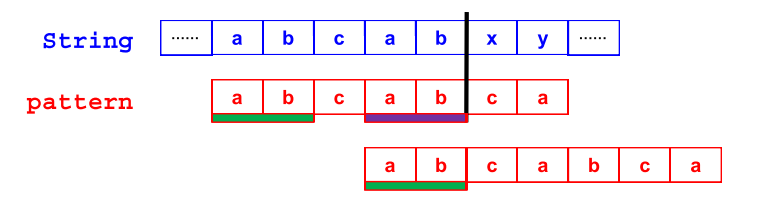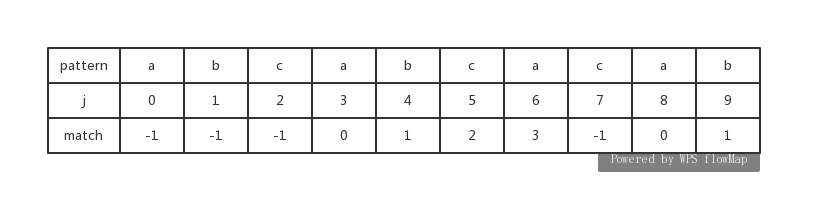# （浙大-19-夏-数据结构)KMP算法剖析数据结构 同时被 2 个专栏收录97 篇文章 2 订阅

KMP算法abcabcabcabcacabxy
3
abcabcacab
cabcabcd
abcabcabcabcacabxyz

abcabcacabxy

#include<Stdio.h>
#include<stdlib.h>
#include<string.h>
#define Notfound -1
int KMP(char string[],char pattern[]);//KMP算法实现
void BuildMatch(char pattern[],int match[]);//match计算
int main()
{
char string[] = "This is a simple example.";
char pattern[] = "simple";
int p = KMP(string,pattern);//KMP算法实现
if(p == Notfound)
else
printf("%s\n",string + p);
return 0;
}
int KMP(char string[],char pattern[]) //T = O(n+m+Tm)
{
int n = strlen(string);//计算位数
int m = strlen(pattern);
int s,p, *match;//匹配数组
if(n < m)
return Notfound;
match = (int *)malloc(sizeof(int)*m);//申请空间
BuildMatch(pattern,match);//创建匹配数组
s = 0;
p = 0;
while( s < n && p < m)
{
if(string[s] == pattern[p])//相等
{
s++;
p++;
}
else if(p > 0)
p = match[p - 1] + 1;
else//第一个字母不相等
s++;
}
return ( p == m ) ? (s - m) : Notfound;//找到该数时，返回match[j]地址;未找到时，返回-1
}
void BuildMatch(char pattern[],int match[])
{
int i,j;
int m = strlen(pattern);//计算位数
match = -1;
for(j = 1;j <= m - 1;j++)
{
i = match[j - 1];
while( (i >= 0) && pattern[i+1] != pattern[j] )//不相等时
i = match[i];
if(pattern[i+1] == pattern[j])//相等时
match[j] = i + 1;
else
match[j] = -1;
}
return ;
}

05-26272
11-30150610-181078
08-09159
10-19554
09-04434
10-18531
05-315675
11-273872
10-18228
02-11248
03-27191
01-223503
02-17188
02-211115
12-19288
06-22276
05-24634
11-132090点击重新获取扫码支付余额充值